## Tuesday, September 29, 2009

Hi all,

Have you ever imagined running optimization algorithms like genetic algorithms or particle swarm optimization on portable devices like cellphones ?

So, let me say that it's possible! First of all, one excellent work on running genetic algorithms on devices like Sony PSP and Symbian Phones was done by Christian Perone, author of the PyEvolve Framework (Python Genetic Algorithms Framework) written all in Python!

His work inspired me to port my old undergraduate project, the particle swarm optimization algorithm implementation in Java to Python! I decided to develop it from scratch and now it's almost complete for its first official release: The PyPSO Toolbox. You can take a look at the project and the source at its official home page repository.

Soon, it will be official available! Until there, i am doing some tests with the framework, correcting some bugs, documenting the source code, etc. But i was wondering here if it's possible to run also the PyPSO framework in the cellphone, like the Symbian Nokia 5800 XpressMusic.

Using the new version of the PyS60, the release 1.9.7, which comes with the new 2.5.1 Python core, I've executed the PyPSO toolbox without problems, and i was very amazed by the good performance of the PSO on the Nokia 5800, the problem that i ran was the minimization of one of the De Jogn's test suite functions, the Sphere function. The function is very simple as you can see its plot below at 3-D chart:I've used particles with 5 dimmensions each with real values between the interval of [-5.12, 5.12], the Constricted Factor and the Global Topology of the PyPSO framework. Also i've set a population size of 30 particles.

After 36 iterations (about 12 seconds), the PSO execution ended with the best fitness of 0.0, representing the optimal solution (the minimum) of the De Jong's Sphere function.

Follow some screenshots of the simulation (click on the pictures to enlarge):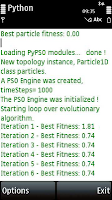How to install PyPSO on the PyS60 ?

1) First, you need to install the PyS60 runtime for your Symbian platform. You can see more information about how to install it here.

2) Then, you must create a directory on your Memory Card or Phone Memory (Depends on where you installed the Python Script Shell), inside the "Python" directory, named "lib" , and inside this directory, you copy the "pypso" folder. The absolute folder structure will be like this:

C:\Python\lib\pypso or E:\Python\lib\pypso

Some features of the framework will not work, like some report Adapters, however, the PSO core is working really great. I've used the PyPSO subversion release 0.11. Since it's not finished yet, you can download from the subversion repository from here.

Here is the source code I've used to minimize the De Jong's Sphere function (Special thanks to Christian Perone who inspired me to do this work) :

`"""PyPsoS60Demo.pyAuthor: Marcel Pinheiro Caraciolocaraciol@gmail.comLicense: GPL3"""BLUE  = 0x0000ffRED   = 0xff0000BLACK = 0x000000WHITE = 0xffffffimport e32, graphics, appuifwprint "Loading PyPSO modules... ",e32.ao_yield()from pypso import Particle1Dfrom pypso import GlobalTopologyfrom pypso import Psofrom pypso import Constsimg = Nonecoords = []graphMode = Falsecanvas  = Nonew = h  = 0print " done !"e32.ao_yield()def handle_redraw(rect): if img is not None:  canvas.blit(img)def sphere(particle): total = 0.0 for value in particle.position:  total += (value ** 2.0)  return total def pso_callback(pso_engine): it = pso_engine.getCurrentStep() best = pso_engine.bestParticle() if graphMode:  img.clear(BLACK)  img.text((5, 15), u"Iteration %d - Best Fitness: %.2f" % (it,best.ownBestFitness), WHITE, font=('normal', 14, appuifw.STYLE_BOLD))  img.line((int(w/2),0,int(w/2),h),WHITE)  img.line((0,int(h/2),w,int(h/2)),WHITE)    cx = int(w/2) / int(best.getParam("rangePosmax"))  cy = int(-h/2) / int(best.getParam("rangePosmax"))    for particle in pso_engine.topology:   img.point([int(cx*particle.position+ (w/2)),int(cy*particle.position+(h/2)),5,5],BLUE,width=4)  img.point([int(cx*best.ownBestPosition+ (w/2)),int(cy*best.ownBestPosition+(h/2)),5,5],RED,width=4)  handle_redraw(()) else:   print "Iteration %d - Best Fitness: %.2f" % (it, best.ownBestFitness) e32.ao_yield()  return Falsedef showBestParticle(best): global graphMode  if graphMode:  img.clear(BLACK)  img.text((5, 15), u"Best particle fitness: %.2f" % best.ownBestFitness, WHITE, font=('normal', 14, appuifw.STYLE_BOLD))  img.line((int(w/2),0,int(w/2),h),WHITE)  img.line((0,int(h/2),w,int(h/2)),WHITE)  cx = int(w/2) / int(best.getParam("rangePosmax"))  cy = int(-h/2) / int(best.getParam("rangePosmax"))  img.point([int(cx*best.ownBestPosition+ (w/2)),int(cy*best.ownBestPosition+(h/2))],RED,width=7)  handle_redraw(())  e32.ao_sleep(4) else:  print "\nBest particle fitness: %.2f" % (best.ownBestFitness,)  if __name__ == "__main__": global graphMode, canvas, w, h  data = appuifw.query(u"Do you want to run on graphics mode ?", "query") graphMode = data or False  if graphMode:  canvas = appuifw.Canvas(redraw_callback=handle_redraw)  appuifw.app.body = canvas  appuifw.app.screen = 'full'  appuifw.app.orientation = 'landscape'  w,h = canvas.size  img = graphics.Image.new((w,h))  img.clear(BLACK)  handle_redraw(())  e32.ao_yield()  #Parameters dimmensions = 5 swarm_size = 30 timeSteps = 100  particleRep = Particle1D.Particle1D(dimmensions) particleRep.setParams(rangePosmin=-5.12, rangePosmax=5.13, rangeVelmin=-5.12, rangeVelmax=5.13, bestFitness= 0.0 ,roundDecimal=2) particleRep.evaluator.set(sphere)  topology = GlobalTopology.GlobalTopology(particleRep) pso = Pso.SimplePSO(topology) pso.setTimeSteps(timeSteps) pso.setPsoType(Consts.psoType["CONSTRICTED"]) pso.terminationCriteria.set(Pso.FitnessScoreCriteria) pso.stepCallback.set(pso_callback) pso.setSwarmSize(swarm_size)  pso.execute()  best = pso.bestParticle() showBestParticle(best) `

To make things more interesting, i've decided to put a simple graphic mode, when the user can choose to see the simulation in graphics mode or console mode. So as soon as you start the PyPsoS60Demo.py script, a dialog will show up to ask which mode the user prefer to see the simulation. You can see the video of the graphics mode running below.

Each point is a particle and they're updating their position through the simulation and since the best optimal solution of the problem is the point (0,0), in the end of the simulation, the particles will be close to the best solution. You can see that the red point is the best particle of all swarm.

You can note at the source code the use of the module "e32" of the PyS60, this is used to process pending events, so we can follow the statistics of the current iteration while it evolves.

I hope you enjoyed this work, the next step is to port the Travelling Salesman Problem to cellphone!

Marcel Pinheiro Caraciolo

## document.write(ultimaFecha);

Hi Folks,

I' am Marcel Pinheiro Caraciolo, master degree candidate at Federal University of Pernambuco (CIN/UFPE) located at Recife - Pernambuco - Brazil. As you can see, i am one of the main authors of the A.I. In Motion Blog (funny but the only one who posts here, but this is not the case!). My interests are about artificial intelligence and mobile computing. How to connect them is my daily work and my master thesis will be specific about data mining algorithms (as you saw in the last posts about data mining) and recommendation systems targeted to Mobile devices. On a next post i will talk further about it.

But, in general, one of my goals at my master degree thesis is to study and apply artificial intelligence and data mining algorithms into mobile computing, solving problems or turning possible those machine learning algorithms to offer their results or run them into small and limited processing devices like cell phones.Let's build real Smart Phones!!

For inspiring our readers to read more about artificial intelligence and mobile computing connected, i've found this article in the internet: "The Artificial Intelligence goes Mobile" An excellent reading for people who wants to inspire themselves and think how the artificial intelligence can be applied at mobile devices. This article was the Introductory remarks of the Artificial Intelligence in Mobile Systems - AIMS2000 Workshop at 2000.
So , stay tuned! More content and tutorials about those topics will be presented here!

Regards,

Marcel Pinheiro Caraciolo

## document.write(ultimaFecha);Hi Folks,

I am Marcel Pinheiro Caraciolo , the main author of this blog!

One of my goals at my master degree thesis is to study and apply artificial intelligence and data mining algorithms into mobile computing, solving problems or turning possible those machine learning algorithms run into small and limited processing devices like cell phones. I've already talked about this topic here.

I'm studying now about recommendation system engines and my plan is to develop a system based on machine learning techniques , data mining to offer specific products and services for mobile users that buys using the mobile phone. Based on mobile technology, the system will be possible to estimate by mobile payment the profile of a specific client and recommend products and services into the mobile device screen, with some profile analysis of the user behavior and consuming of products acquired using the mobile phone. We can resume the system features into 3 key points:

(a) Estimate the profile of clients and sellers to make recommendations of services and products.
(b) The automatic discovery of knowledge with induction of rules that explain the operational behavior on cellphones.

(c) The investigation of profiles on any characteristics in the mobile transactions (region, age, gender, consume, salary, habits, etc.)

During those months we will study a lot about data mining algorithms, mobile computing and recommender systems. Stay tuned!

Marcel Pinheiro Caraciolo

## Monday, September 28, 2009

In this article, we will explore one of the basic steps in the knowledge discovery process, "Data Preprocessing", an important step that can be considered as a fundamental building block of data mining. The process of preprocessing has many steps, but can be summarized as the extraction, transformation and loading of the data. To be more precise modifying the source data into a different format which:

(a) enables data mining algorithms to be applied easily

(b) improves the effectiveness and the performance of the mining algorithms

(c) represents the data in easily and understandable format for both humans and machines

(d) supports faster data retrieval from databases

(e) makes the data suitable for a specific analysis to be performed.

The real world data can be considered extremely complicated to interpret without Data Preprocessing. I am going to explain this through a simple example based on Normalization. For people who come from database background this Normalization is completely different from 1st, 2nd and 3rd form of normalization used in the relational database design. We are talking about another type of normalization, and it's related to data preprocessing technique. To see how a simple data preprocessing technique could improve the effectiveness of analysis in orders of magnitude, let's move on further and talk about euclidian distance and how it's can be used to evaluate similarities between samples of data.

Euclidian Distance

Consider two points in a two-dimensional space (p1,p2) and (q1,q2) , the distance between these two points is given by the formula shown at the Figure 01.Figure 01. Euclidean Distance Measure for 2-D Points

This is called the Euclidian Distance between two points. The same concept can be extended easily to multidimensional space. If the points are (p1,p2,p3,p4,...) and (q1,q2,q3,q4,...), the distance between the points is given by the formula (Figure 02).Figure 02. Euclidean Distance for Multi-Dimensional Points

Now, I am going to introduce a sample data set of mobile profile users details. The task is to find the mobile users who have similar profiles, that is, that has similar use of the phone based on the call and SMS logs.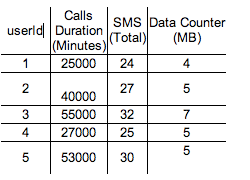Table 01. Example Data Set (Mobile users profiles during one month)

In this data set, the first column is an unique identifier and the rest of the columns contain information. Ignoring the ID attribute, if we consider each column (attribute) as a dimension we can assume that each mobile user is represented by a point in 3-D space. The good thing is that we can calculate the distance between each of these points. The distance between points 1 and 2, (user 1 and 2) can be calculated as below: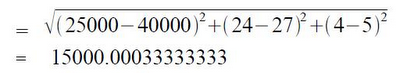Figure 03. Euclidean Distance between users 01 and 02.

Look at the data set above, through examination we figure out that the user id 1 and 4 are users with almost similar profiles, that is, use their phones very similar. So in the three dimensional space the distance between them should be less, that is, these two points should be very close.

The following table (Figure 04) gives the euclidean distance calculation for each user with the other users in our given data set.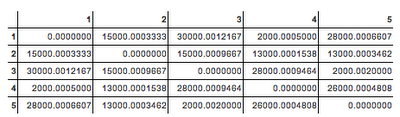Figure 04. Euclidean Distance Matrix for all users

We can see from the above list that the distance between the user 1 and 4 is 2000.00, which is less when compared between userId 1 and other users. So our interpretation seems to be correct. However, an alert reader would have noticed a significant observation here. If you see the list again, you can see that the euclidian distance values are very close to the differences in duration calls. Which this means ?

See this, the difference between the duration calls of users 1 and 4 is abs(25000 - 27000) = 2000, and the euclidean distance between one and four is 2000.30303! So it looks like this approach seems to be flawed. The euclidean distances are dominated by the duration calls amount. So guess you can't rely on euclidean distance to find mobile users with similar profile.

How do we get ourselves out of this problem ? We do not want the duration calls attribute to dominate the euclidean distance calculation. We can achieve this by applying one of the Data Preprocessing techniques called Normalization over the data set.

What is Normalization ?
The attribute data is scaled to fit into a specific range. There are many types of normalization available, we will see one technique called Min-Max Normalization. Min-Max Normalization transforms a value A to B which fits in the range [C,D]. It is given by the formula below (Figure 05):Figure 05. Min-Max Normalization Formula

Consider the example below, the duration calls value is 50000, we want to transform this in to the range [0.0 , 1.0], so first we find the maximum value of duration calls which is 55000 and the minimum value of duration calls, 25000, them the new scaled value for 50000 will be: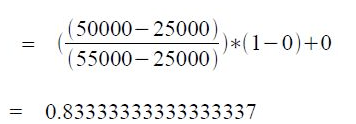Figure 06. Min-Max for Duration Calls Attribute from the User 01

Now let's apply the normalization technique to all the three attributes in our data set. Consider the following maximum and minimum values:

Max duration calls = 55001
Min duration calls = 24999
Min SMS = 23
Max SMS = 33
Max consume data = 8
Min consume data = 3

The attributes need to be scaled to fit in the range [0.0 , 1.0]. Applying the min-max normalization formula above, we get the normalized data set as given below (Figure 07):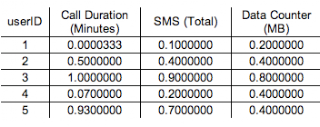Figure 07. Data set after Normalization

Now given the new data set all normalized, We will calculate the euclidean distances for each employee with the other employees. It's given in the table below (Figure 08):

Now compare the euclidean distance calculation before and after normalization. We can see that the distances are no more dominated by the duration calls attribute and they make more sense now. The number of messages and data consumed now also contribute to the distance calculation. You can see how the normalization technique and data preprocessing can really help get useful and right information about your data before applying some machine learning or data mining algorithm.

To conclude this tutorial, it's important to notice that there are many measures similar to euclidean distance which can be used to calculate the similarity between two records such as Pearson coefficient, Tanimoto Coefficient, etc. You can try replacing euclidean distance with any of these measures and experiment.

You can read more on these measures in the following link. Take a special look at other normalization techniques such as Xˆ2 (X Square) Normalization and decimal scaling which are also worth trying.

Any doubts or suggestions,

Please make yourself welcome to give!

Marcel Pinheiro Caraciolo

## Sunday, September 27, 2009I was reading some blogs today, when i came into this post at Gizmodo, about the the proof concept of this tablet invented by Apple in 1987! Amazing, how they at that time was thinking about multi-touch features, speech-text, artificial intelligence, tablets, video and voice talk by over-the-air communication, etc. Even this is only a simulated demonstration of the tablet, the Knowledge Navigator of Apple, it would be make people atonished if it launched these days.

But it's really cool, the artificial intelligence shown in this device, like some kind of Star Trek tech, you can see the demonstration at this video provided by Apple in 1987:

Let's see if this kind of device will be presented in a reality soon... Is there already technology to have this kind of interaction between human and machine ?

Source: Gizmodo

## Saturday, September 19, 2009

Hi all,

In this article we will continue our studies about Data Mining algorithms. Now, i will present a supervised learning algorithm called Bayesian Classification. As same as the previous articles presented in this blog, a simple example of the algorithm will be presented which can be executed with Python Interpreter.

The Algorithm

The Bayesian classification algorithm is called with this name because is based on the Bayes' probability theorem. It's known also by Naïve Bayes classification rule or only by Bayesian Classifier.

The algorithm aims to predict the class membership probabilities, such as the probability that a given tuple or pattern belongs to a particular class,that is, predict the most probable class that the pattern belongs to. This type of prediction is called statistical classification, which is totally based on probabilities.

This classification also is called a simple or Naïve, because it assumes that the effect of an atribute value on a given class is independent of the values of the other attributes. This assumption is called class conditional independence and it's made to simplify the computations involved.

Furthermore about attributes, it's important to notice that the Bayesian classifier gets better results when the attribute values are categorical instead of continuous-valued . Maybe this will be more clear at the example that will be shown soon.

Other characteristic of the algorithm is that it requires data set already classified, that is, a set of patterns and their associated class labels. Based on this data set, called also 'training data set ' , the algorithm receives as input a new pattern (unknown data), that is, data patterns for which the class label is not known, and returns as output the class which the probability calculated for this data is maximum in accordance to probabilistic calculations. Different from the K-means algorithm seen in the previous article, the Bayesian classifier doesn't need a metric to compare the 'distance' between the instances and neither classifies the unknown pattern automatically, since it's necessary a data set already classified (training set). Because of this requirement, the Bayesian Classification algorithm is considered a supervised data mining algorithm.

To see how the algorithm works, let's resume it with the following four steps:

Step 01: Probabilities classes evaluation (Class Prior Probability).

In this step, each class of the training set has its probability calculated. In most of times, we only work with two classes, for instance, one class shows if a certain consumer buys or not a product based on his demographic characteristics. The calculation is done by dividing the number of patterns of a specific class by total number of patterns of the training set.

Step 02: Probabilities evaluation of the training set

Now, each value of each attribute of the data of the training set has your probability calculated for each possible class. This step is where occurs the most consuming time computational processing of the algorithm, since depending on the number of attributes, classes and patterns of the training set, it's possible that many calculations must be done before get some results (probabilities).

It's important to notice that this calculation depends totally on the attribute values of the unknown sample data, that is, the sample that you desire to predict the class label. Supposing that there are k classes in the test set and m attributes in the test set, it must be necessary to calculate k x m probabilities.

Step 03: Evaluate the probabilities of the unknown data.

In this step, the probabilities calculated for the patterns of the unknown data of the same class are multiplied. Thus, the result obtained is multiplied by the probability class calculated at the Step 01.

With the probabilities of each class calculated, then check which class has the maximum value for the probability of the unknown data. The algorithm ends returning the class with the probability that has the maximum value (the predicted class) for the unknown data.

Further information about Bayesian classification can be found at the links below:

http://en.wikipedia.org/wiki/Na%C3%AFve_Bayes
http://www.devmedia.com.br/articles/viewcomp.asp?comp=2637

Now, let's see a practical example of the use of Simple Bayesian Classification algorithm with the probabilities evaluation.

Example of the Algorithm

In this example, let's consider that a bank loans officer wants to predict if the client will be a bank defaulter or not. For this, the bank must consider his historical client profiles and some attributes. To make easy the comprehension of the scenario and the data model, let's use a training set with only 15 rows and 4 columns (attributes). The Figure 01 shows the training set that will be used in this example.Figure 01. The historic client profiles (Training Set).

The attributes shown at the Figure 01 are described as below:

CLIENT_ID: This column has an unique integer sequential identifier. For the algorithm this attribute is optional, but it may help to organize the rows of the data set.

GENDER : This attribute identifies the gender of the client. The values allowed are only MALE or FEMALE.

MARITAL_STATUS: This attribute brings information about the marital status of the client. It can be only the values MARRIED or SINGLE.

EDUCATION: This attribute brings the information about the education level of the client. It can assume only four different values: HIGHSCHOOL_INCOMPLETE , HIGHSCHOOL_COMPLETE, GRADUATION_INCOMPLETE and GRADUATION_COMPLETE.

INCOMES: This attributes refers to the earnings of the client. It can only has the values: ONE_MIMINUM_SALARY, TWO_MINIMUM_SALARIES and UPPER_THREE_MINIMUM_SALARIES.

DEFAULTER: This column represents the classification label attribute of the patterns. In this example the classification shows if the client is bank defaulter, that is DEFAULTER=YES, or the client is not bank defaulter, that is, DEFAULTER=NO. To clarify the visualisation, the clients of the training set that are defaulters were marked in red and clients that aren't defaulters are marked in blue.

Let's execute the Bayesian Classification to a given unknown pattern. Based on the data shown at the Figure 01, the target is to predict the class label (DEFAULTER) of this new client shown at the Figure 02 by using the Bayesian Classifier.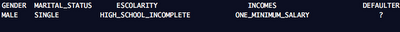Figure 02. The new Client to be classified as DEFAULTER or NOT DEFAULTER

Step 01: The Evaluation of the classes probabilities.

There are only two classes, one that shows the client is bank defaulter (DEFAULTER= YES) and another that points the client is not bank defaulter (DEFAULTER= NO). Calculating the probabilities of the classes, we have:

Probability DEFAULTER= YES : 4/15 = 0,2667

Probability DEFAULTER= NO: 11/15 = 0,7334

Step 02: Calculate the probabilities of the training set.

For the first attribute of the unknown data GENDER=MALE, let's calculate the probability of DEFAULTER=YES:

Probability of GENDER=MALE and INADIPLENT=YES : 2/4 = 0,5

And for the case where the client is male and is not defaulter, we have:

Probability GENDER=MALE and DEFAULTER=NO: 4/11 = 0,3636

For the rest of the attribute values of the data set, we have:

Probability of MARITAL_STATUS =SINGLE and DEFAULTER=YES: 1/4 = 0,25
Probability of MARITAL_STATUS=SINGLE and DEFAULTER=NO: 6/11 = 0,5455

Probability of EDUCATION= HIGHSCHOOL_INCOMPLETE an DEFAULTER=YES: 1/4 = 0,25
Probability of EDUCATION= HIGHSCHOOL_INCOMPLETE and DEFAULTER=NO: 4/11 = 0,3636

Probability of INCOMES= ONE_MIMINUM_SALARY and DEFAULTER=YES: 1/4 = 0,25
Probability of INCOMES= ONE_MIMINUM_SALARY and DEFAULTER=NO: 4/11 = 0,3636

Step 03: Calculate the probability of the unknown data.

Multiplying the probabilities of the unknown data for the case of DEFAULTER=YES by the priori probability of DEFAULTER calculated at the Step 01, we have:

0,5 x 0,25 x 0,25 x 0,25 x 0,2667 = 0,0021

Multiplying the probabilities of the unknown data for the case of DEFAULTER= NO by the probability of NOT DEFAULTER calculated at the Step 01, we have:

0,3636 x 0,5455 x 0,3636 x 0,3636 x 0,7334 = 0,0192

As 0,0192 > 0,0021, the algorithm classifies the unkown pattern as INADIPLENT=NO, that is, this new client has higher probability of not becoming a bank defaulter than becoming one, based on the previous data (training set) and the Bayesian classification.

To help classifying those clients, let's use a implementation of the Bayesian Classification algorithm that will work with only many attributes that has nominal (categorical) values. This implementation was written with Python Script 'bayesian_classify.py' .

>>> python bayesian_classify.py 'C:\dataset.txt' 'DEFAULTER' 'MALE;SINGLE;HIGHSCHOOL_INCOMPLETE;ONE_MINIMUM_SALARY' 1

Figure 03. The bayesian_classify.py call

The first parameter that must be passed as argument of the script is the data set file path. The second parameter must indicate the list of columns used at the classification, all then splitted by comma and at one string. The third parameter shows the column that has the classifications. The fourth parameter must receive the unknown data pattern values list split by comma and in the same order of the attributes passed in the second parameter. The Figure 03 shows the call of the script at the console based on the example shown above.

The Script has one more parameter. If this parameter is passed as 0, the script returns all probabilities of each class. If the parameter is passed as 1, the script returns only the classification of the unknown data. The Figure 04 shows the result of the call of the script presented at the Figure 03.

>>> DEFAULTER=NO
Figure 03. Execution of the bayesian_classifier.py returning the classification.

Therefore, it must have to be considered some observations before using the Bayesian Classifier. It's necessaty that the training set must be correct and consistent, since one line that presents some wrong value can compromise the final result. Other drawback of the algorithm is when there is missing value in the attribute, so the probability is assigned to 0, which makes difficult to give the correct classification of certain samples. Anyway, some techniques have been presented to go around these problems, but it's not the scope of this article now.